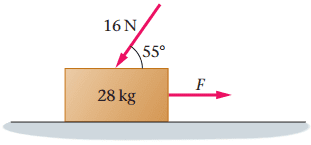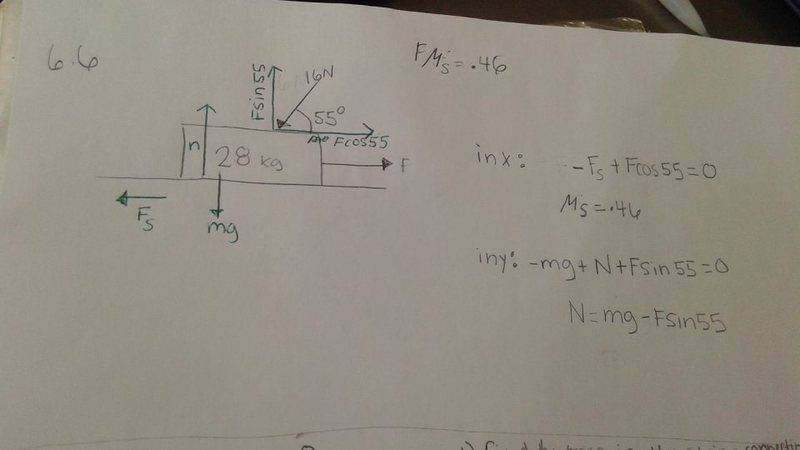# What is the minimum horizontal force?

## Homework Statement

What is the minimum horizontal force F needed to make the box start moving in (Figure 1) ? The coefficients of kinetic and static friction between the box and the floor are 0.25 and 0.46, respectively.Fs= mu * N

## The Attempt at a Solution

So I know that the normal force is 432 N because of the additional force acting on the 28kg object. The angle is throwing me off a little bit. Since I am only finding the horizontal force would I only take into account cos(55)?

gneill
Mentor
The 16 N force will have both horizontal and vertical components. Make a free body diagram that includes the 16 N force's components.

Doc Al
Mentor
So I know that the normal force is 432 N because of the additional force acting on the 28kg object.
Rethink that.

CWatters
Homework Helper
Gold Member
So I know that the normal force is 432 N..

It looks like you did..

(28*9.81)+(16*9.81) = 432

That's not correct for two reasons.

1) The 16N is a force in Newtons already so no need to multiply by g.
2) It's acting at an angle so you only the vertical component contributes to the Normal force.

The 16 N force will have both horizontal and vertical components. Make a free body diagram that includes the 16 N force's components.
Would the F pointing in the right direction be the static friction. or is that different?

doing the free body diagram I got
in x direction: +F-Fs+16cos(55)=0
Fs=.46
in y direction: +N-mg+16sin(55)=0

am I correct?

gneill
Mentor
doing the free body diagram I got
in x direction: +F-Fs+16cos(55)
No. The 16 N force is directed down and to the left, so it can't have a component that points to the right. And the above isn't an equation. There should be an "=" sign for it to be an equation.
Fs=.46
0.46 what? If Fs is meant to be a force then it must have units of force. And 0.46 just looks like the static friction coefficient, not the static friction itself.
in y direction: +N-mg+16sin(55)
Again, no "=" so it's not an equation. But no, gravity and the vertical component of the 16 N force should have the same direction, right? Again, the 16 N force is pointing down and left. So its components can only be down and left.
am I correct?[/QUOTE]
Not this time.

Can you post an image of your FBD?

CWatters
Homework Helper
Gold Member
Would the F pointing in the right direction be the static friction. or is that different?

F is a second applied force not the friction force. They are asking how big F must be for the box to move to the right. In that case which direction will friction act?

Post your free body diagram as others have suggested.

F is a second applied force not the friction force. They are asking how big F must be for the box to move to the right. In that case which direction will friction act?

Post your free body diagram as others have suggested.
how can I upload a picture?

gneill
Mentor
how can I upload a picture?
You can upload an image file from your computer using the UPLOAD button at the bottom right of edit window. You can also copy/paste images directly in many cases.

I uploaded a picture both methods. Are you able to see it?gneill
Mentor
I uploaded a picture both methods. Are you able to see it?
Yes, the attached image is fine.

Your force components for the 16 N force are both in the wrong direction. They should sum to give the original force. Your indicated component directions would sum to give a force directed upwards and to the right, exactly the opposite of the original 16 N force vector.

You've also labeled the 16 N force components as Fsin 55 and F cos55, and also labeled the horizontally applied force F. That's confusing because they are not related.

inx
Yes, the attached image is fine.

Your force components for the 16 N force are both in the wrong direction. They should sum to give the original force. Your indicated component directions would sum to give a force directed upwards and to the right, exactly the opposite of the original 16 N force vector.
okay so i get why 16 N would be - in the y direction. But why would it be downward and to the left? if that is what you are saying.

gneill
Mentor
inx

okay so i get why 16 N would be - in the y direction. But why would it be downward and to the left? if that is what you are saying.
Look at the direction its arrow is pointing. The 16 N force is directed down and to the left on the image . It's pressing down on the block and push it to the left.

okay. I found the Normal force which is equal to 288N. Now would I use the formula Fs=Mu N?
Fs=(.46)(288N)= 132 N

gneill
Mentor
okay. I found the Normal force which is equal to 288N. Now would I use the formula Fs=Mu N?
Fs=(.46)(288N)= 132 N

That's the idea. I think you meant to write: ##F_s = μF_N##? Your result for the maximum static friction force is good.

Note that you can access a menu of Greek letters and math symbols if you clock on the ##\Sigma## icon in the edit panel top menu bar.

Also a suggestion: It's okay to round results for presentation, but don't round calculated values in intermediate steps. Any value that will be used for further calculations shouldn't be rounded, otherwise what's known as rounding and truncation error will accumulate over successive calculations, eventually creeping into your significant figures. It's usually sufficient to keep a few extra decimal places for values that you need to "remember" by writing them down, to guard against this happening.